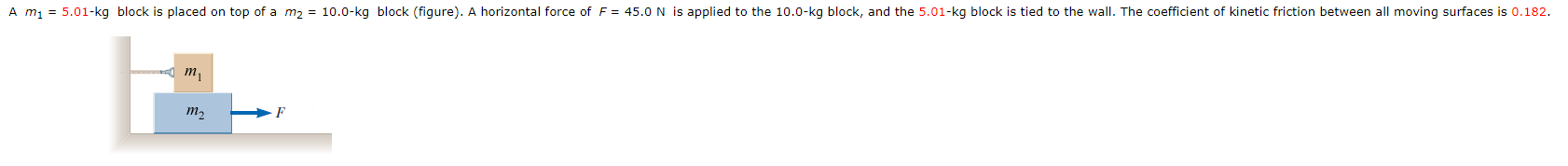# ontopofam2-10.0-kgblock(figure).Ahorizontalforce of F 45.0 N is applied to the 10.0-kg block, and tkg block, and the 5.01-kg block is tied to theA m1wallI. The coefficient of kinetic friction between allmoving surfaces is 0.182.m,

Question
12 views

A m1 = 5.01-kg block is placed on top of a m2 = 10.0-kg block (figure). horizontal force of F = 45.0 N is applied to the 10.0-kg block, and the 5.01-kg block is tied to the wall. The coefficient of kinetic friction between all moving surfaces is 0.182.
(a)Draw a free-body diagram for each block and identify the action–reaction forces between the blocks.
(b) Determine the tension in the string in magnitude of newtons.
(c) Determine the magnitude of the acceleration of the 10.0-kg block.help_outlineImage Transcriptioncloseon top of a m2 - 10.0-kg block (figure). A horizontal forc e of F 45.0 N is applied to the 10.0-kg block, and t kg block, and the 5.01-kg block is tied to the A m1 wall I. The coefficient of kinetic friction between all moving surfaces is 0.182. m, fullscreen
check_circle

Step 1

Given,

Mass of object 1, m1 = 5.01 kg

Mass of object 2, m2 = 10.0 kg

Acceleration of gravity, g = 9.81 m/s2

Force on object 2, F = 45.0 N

Coefficient of kinetic friction, µ = 0.182

Step 2

Free body diagram of blocks.

Step 3

From the equilibrium forces on the objec...

### Want to see the full answer?

See Solution

#### Want to see this answer and more?

Solutions are written by subject experts who are available 24/7. Questions are typically answered within 1 hour.*

See Solution
*Response times may vary by subject and question.
Tagged in

### Kinematics